## The Hubble Space Telescope has a mass of 1.16*10^ 4 kg and orbits the Earth at an altitude of 5.68 * 10 ^ 5 above Earth’s surface. Relative

Question

The Hubble Space Telescope has a mass of 1.16*10^ 4 kg and orbits the Earth at an altitude of 5.68 * 10 ^ 5 above Earth’s surface. Relative to infinitydetermine the potential energy the telescope at this location. Would the formula be Ep=-Gm1m2/r or positive G since it’s relative to infinity

in progress 0
2 months 2021-07-31T19:33:34+00:00 1 Answers 2 views 0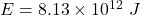Explanation:

Given that,

The mass of a Hubble Space Telescope,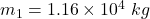It orbits the Earth at an altitude of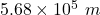We need to find the potential energy the telescope at this location. The formula for potential energy is given by :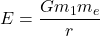Where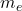is the mass of Earth

Put all the values,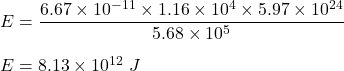So, the potential energy of the telescope is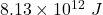.